# RD Sharma Solutions for Class 9 Maths Chapter 3 Rationalisation Exercise 3.2

RD Sharma Class 9 Mathematics Chapter 3 Exercise 3.2 Rationalisation is provided here. Our experts have designed RD Sharma Class 9 chapter 3 solutions in accordance with CBSE syllabus for class 9. Learning this chapter will also help the students to score good marks in the examination. Students can download RD Sharma Solutions for Class 9 Chapter 3 Exercise 3.2 by clicking on the link below.

## Download PDF of RD Sharma Solutions for Class 9 Maths Chapter 3 Rationalisation Exercise 3.2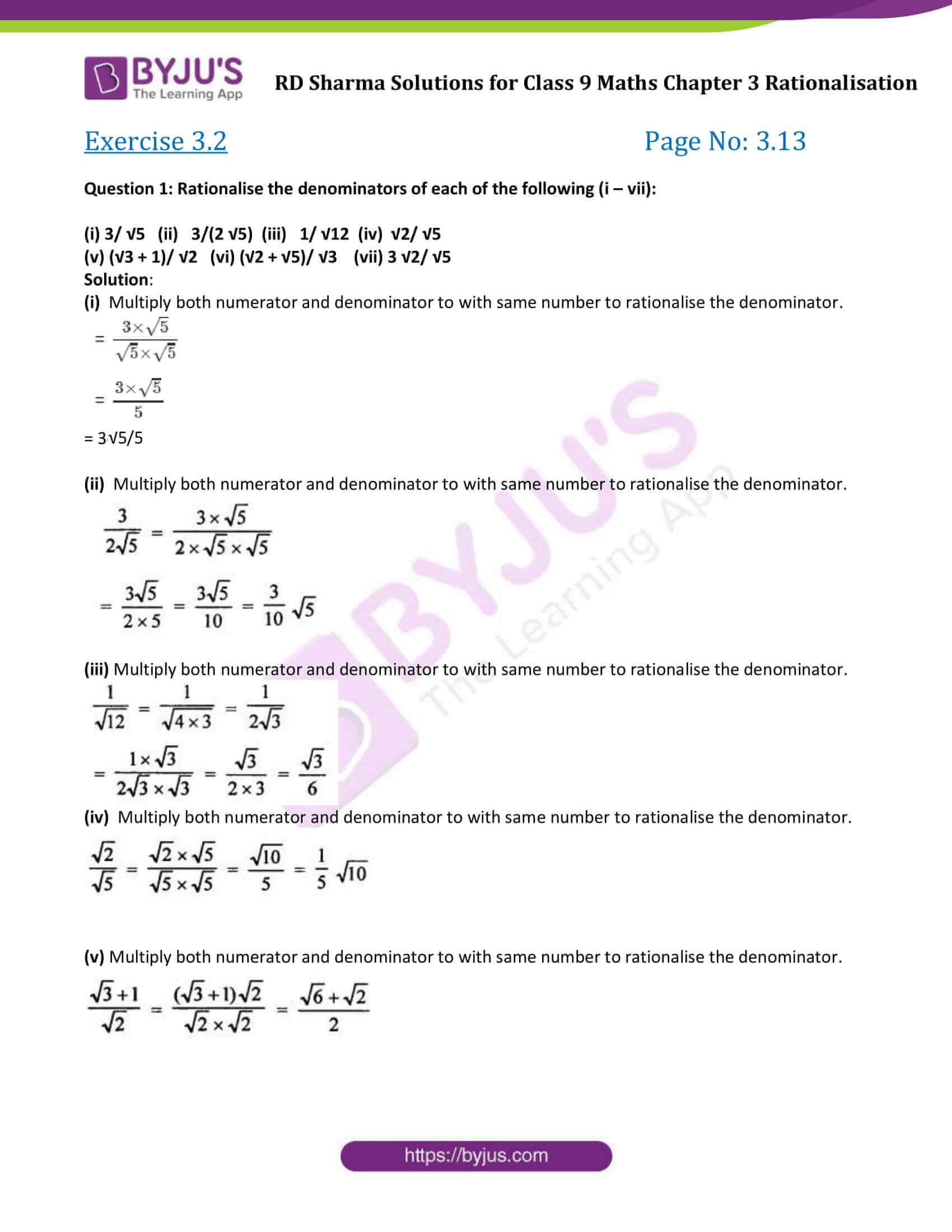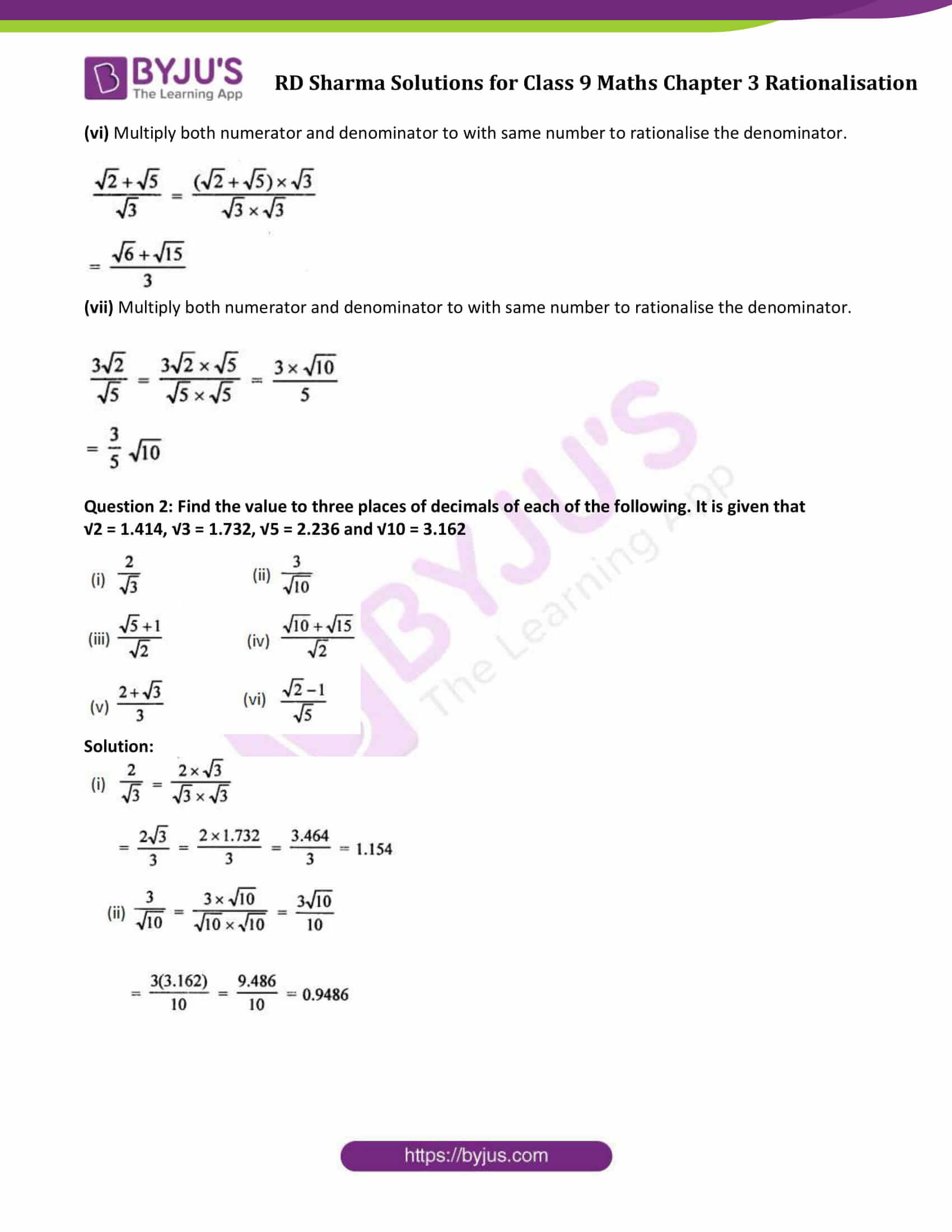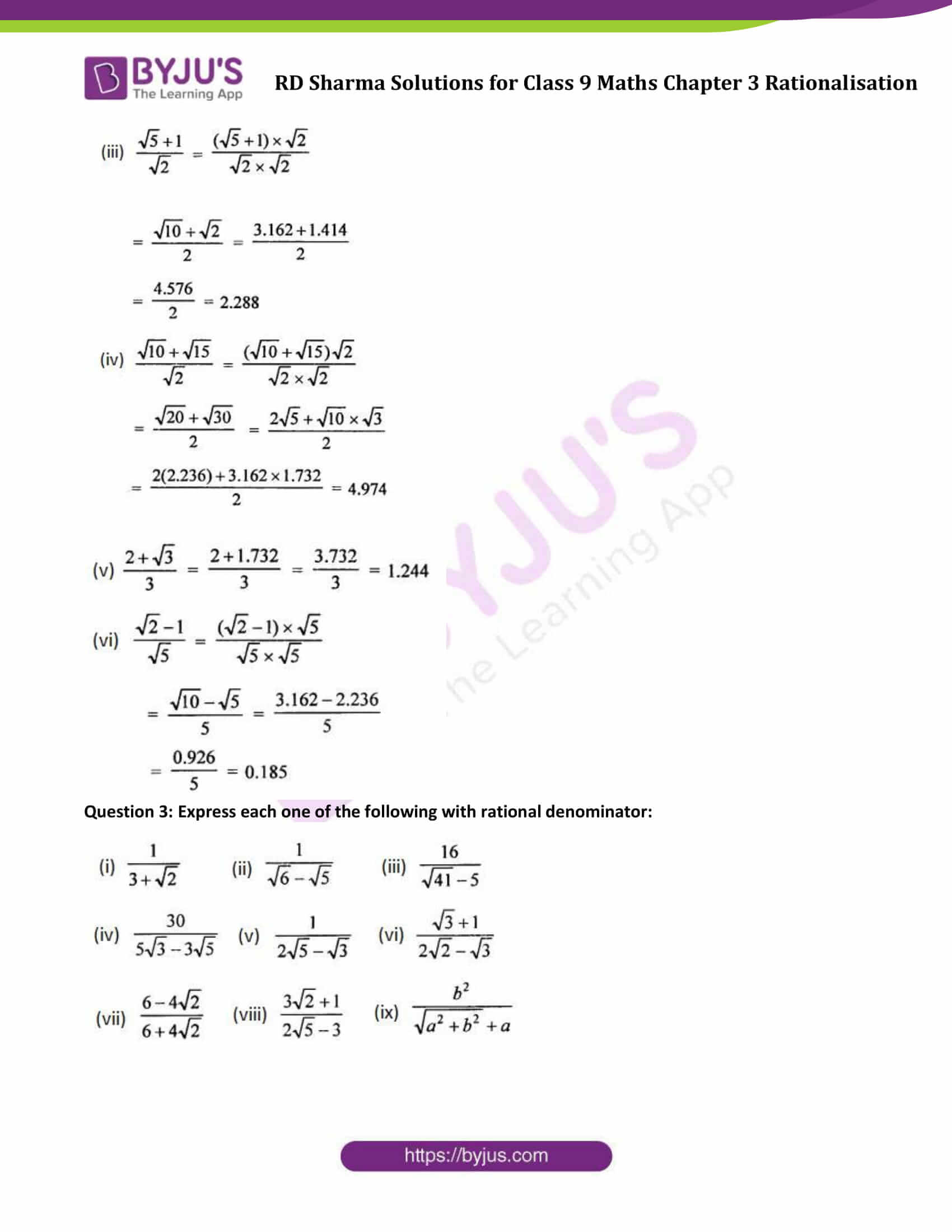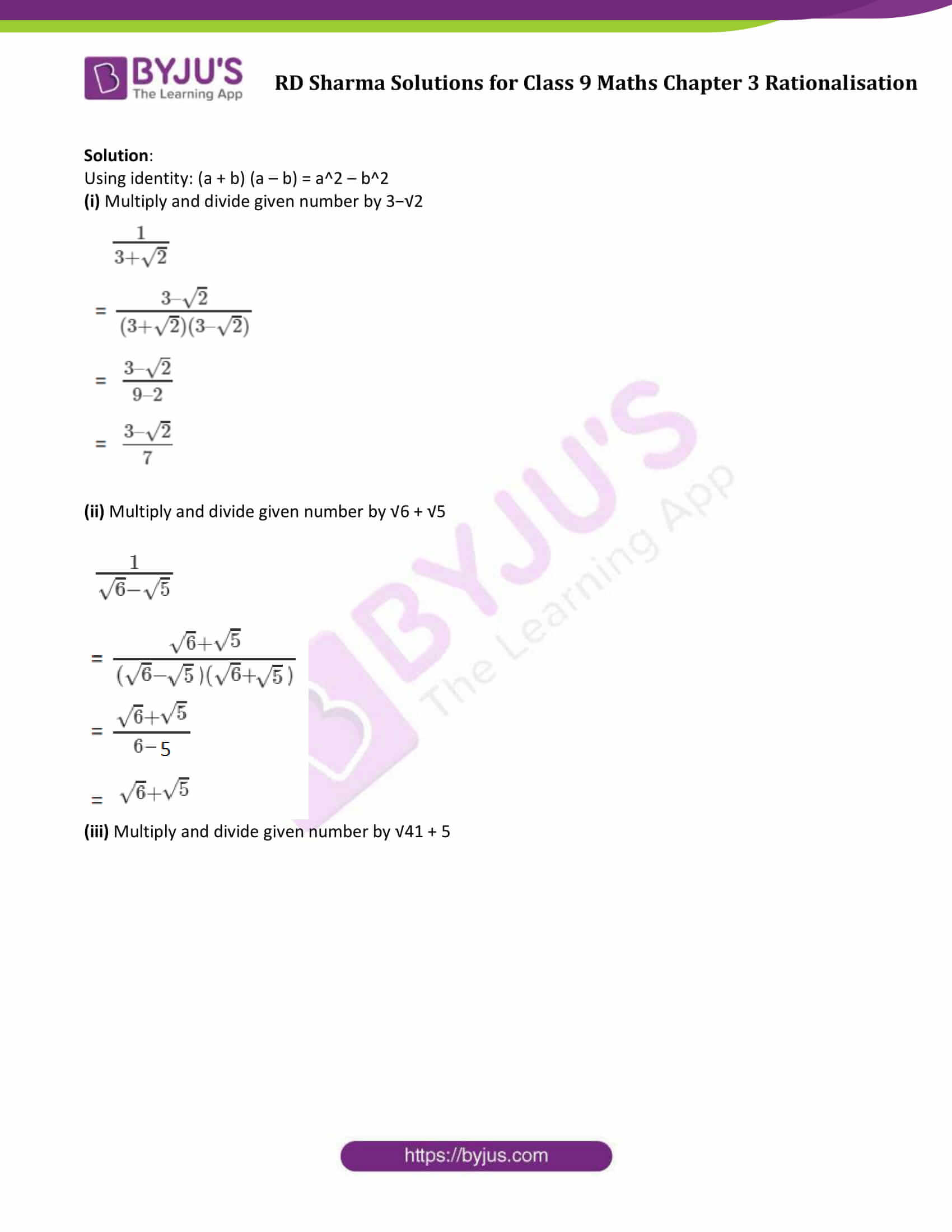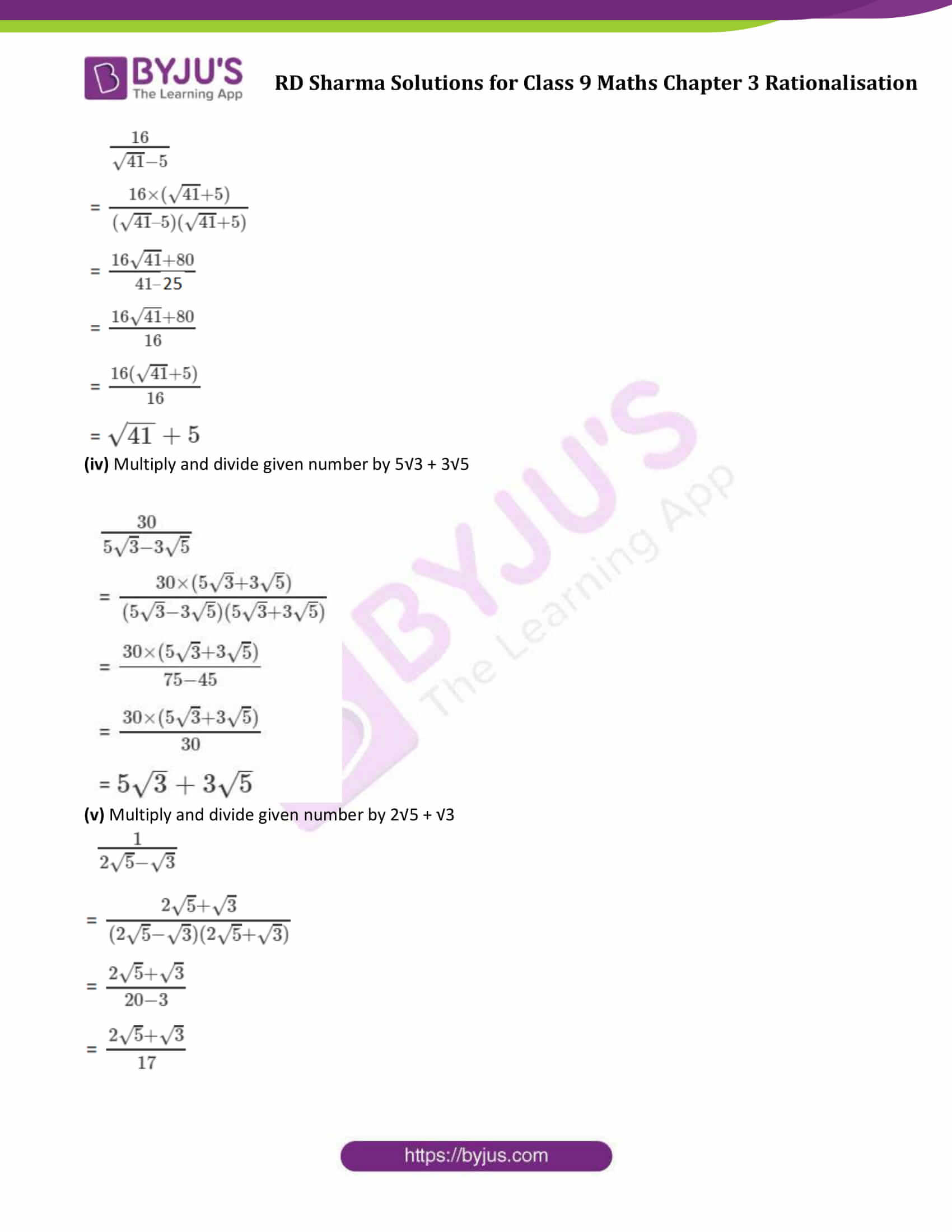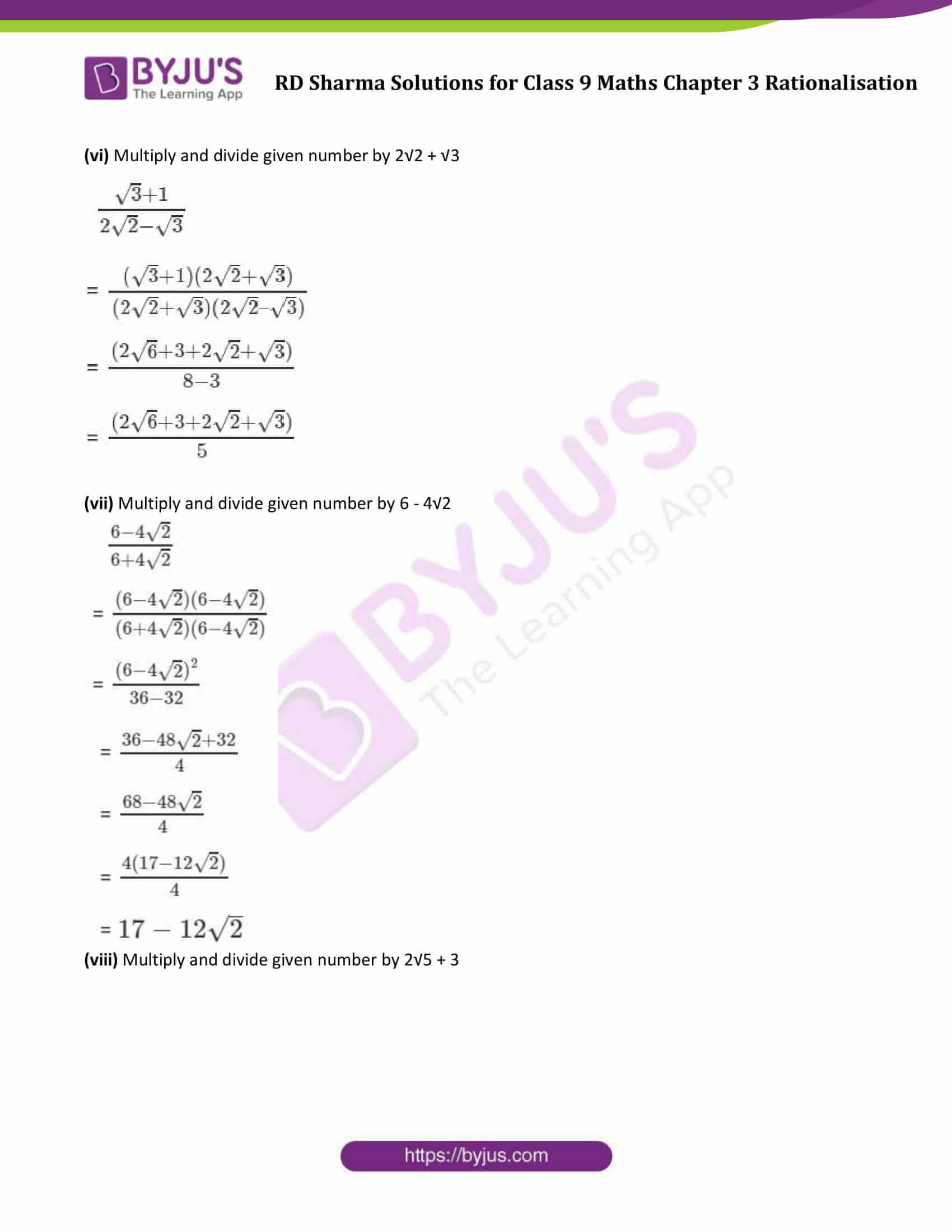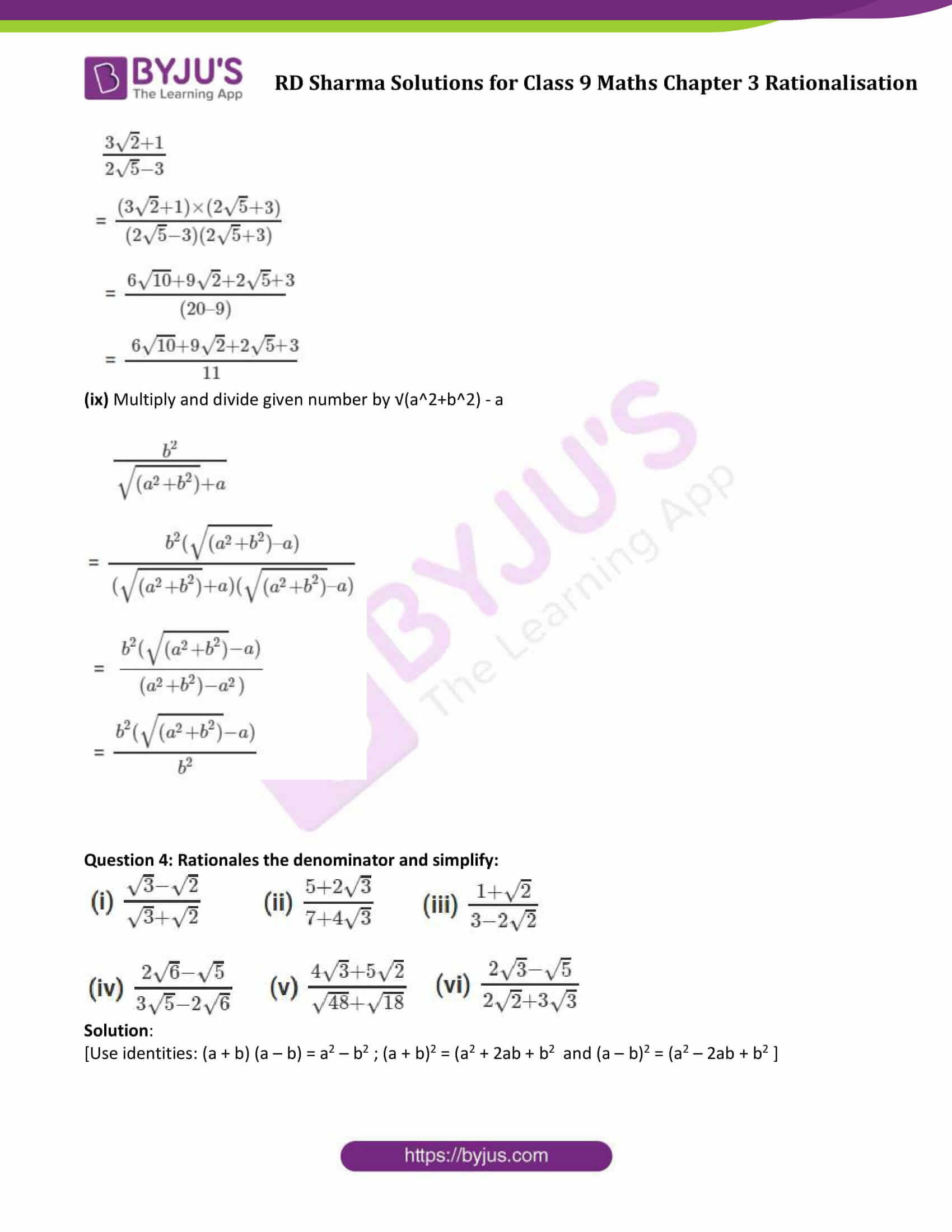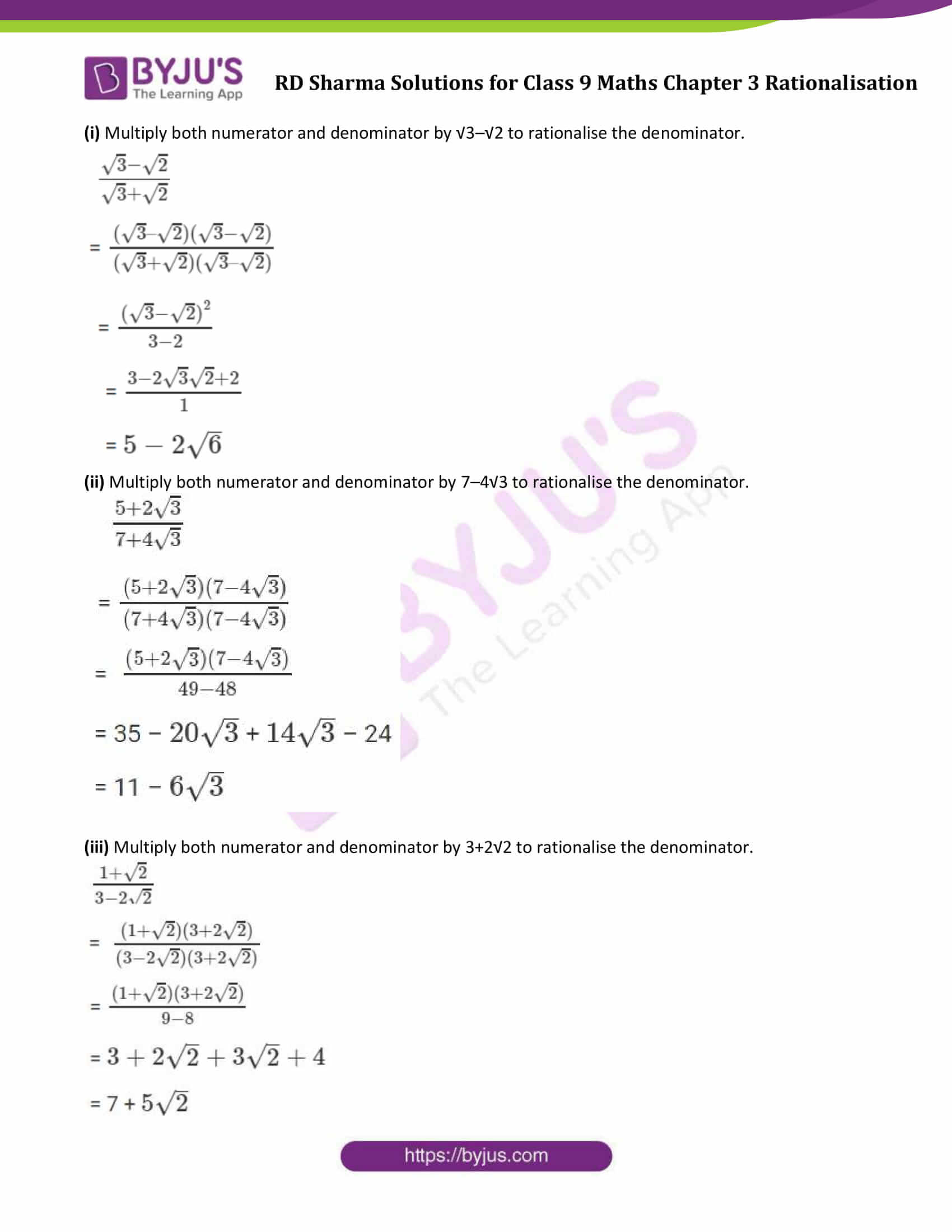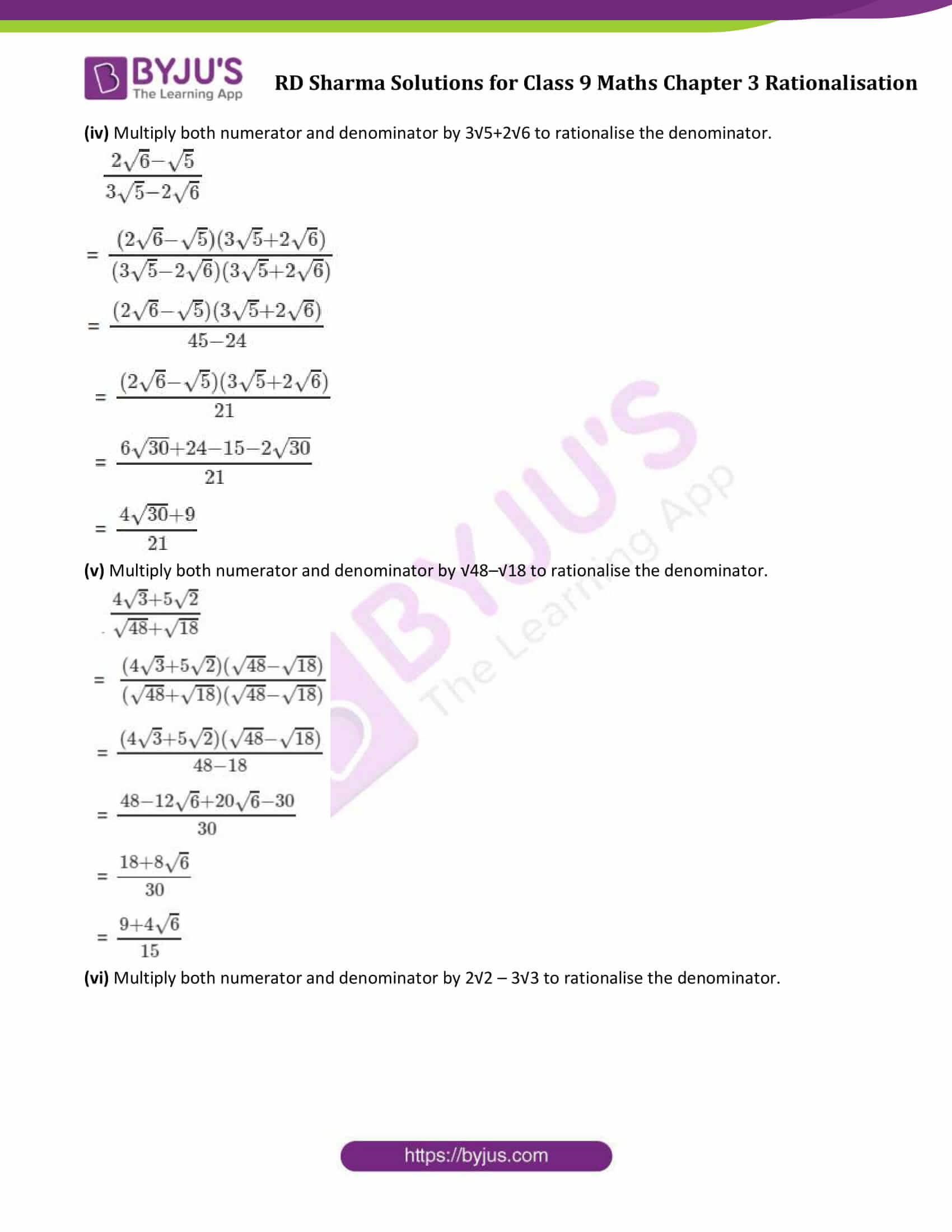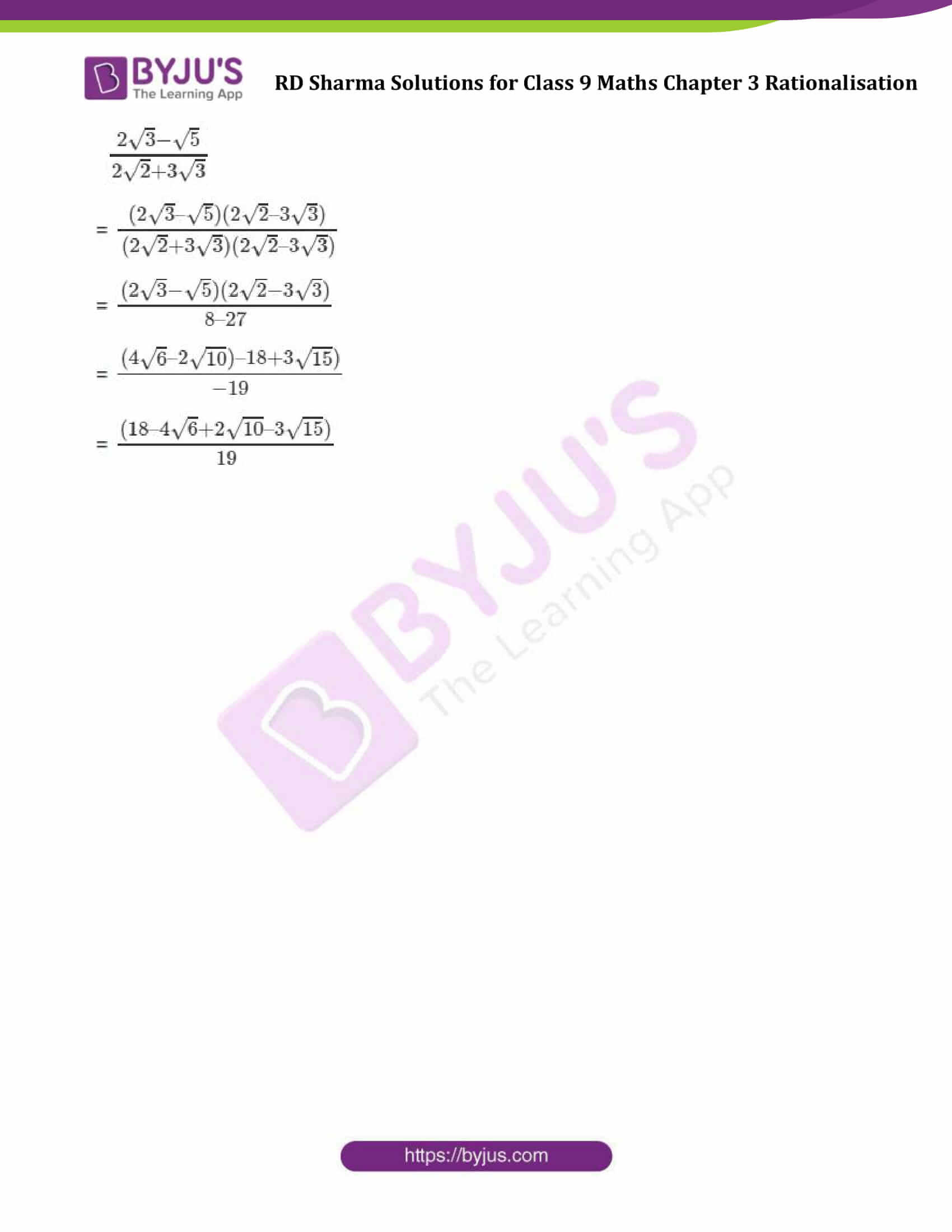### Access Answers to Maths RD Sharma Solutions for Class 9 Chapter 3 Rationalisation Exercise 3.2

Question 1: Rationalise the denominators of each of the following (i – vii):

(i) 3/ √5 (ii) 3/(2 √5) (iii) 1/ √12 (iv) √2/ √5

(v) (√3 + 1)/ √2 (vi) (√2 + √5)/ √3 (vii) 3 √2/ √5

Solution:

(i) Multiply both numerator and denominator to with same number to rationalise the denominator.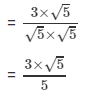= 3√5/5

(ii) Multiply both numerator and denominator to with same number to rationalise the denominator.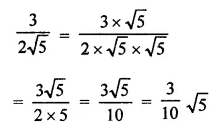(iii) Multiply both numerator and denominator to with same number to rationalise the denominator.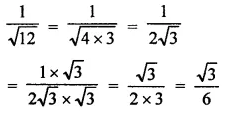(iv) Multiply both numerator and denominator to with same number to rationalise the denominator.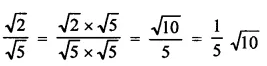(v) Multiply both numerator and denominator to with same number to rationalise the denominator.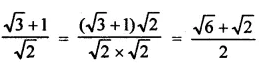(vi) Multiply both numerator and denominator to with same number to rationalise the denominator.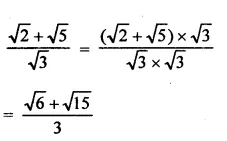(vii) Multiply both numerator and denominator to with same number to rationalise the denominator.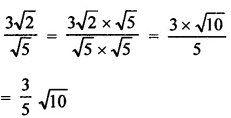Question 2: Find the value to three places of decimals of each of the following. It is given that

√2 = 1.414, √3 = 1.732, √5 = 2.236 and √10 = 3.162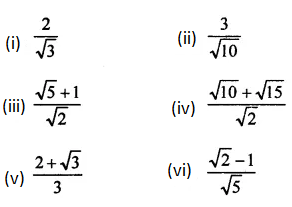Solution: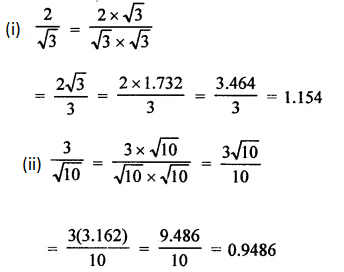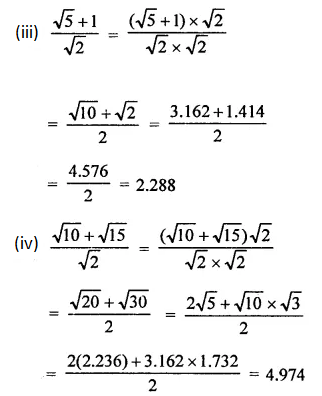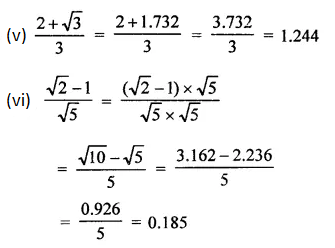Question 3: Express each one of the following with rational denominator: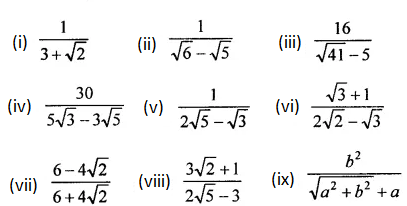Solution:

Using identity: (a + b) (a – b) = a2 – b2

(i) Multiply and divide given number by 3−√2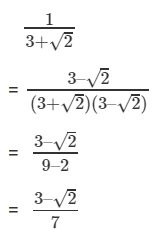(ii) Multiply and divide given number by √6 + √5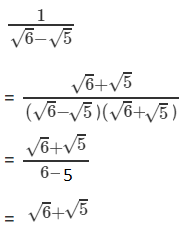(iii) Multiply and divide given number by √41 + 5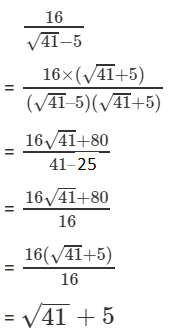(iv) Multiply and divide given number by 5√3 + 3√5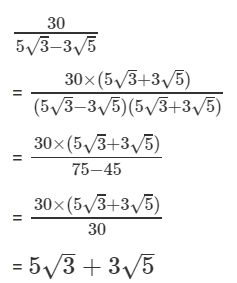(v) Multiply and divide given number by 2√5 + √3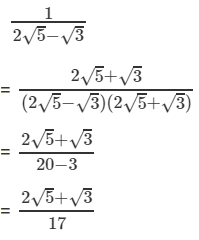(vi) Multiply and divide given number by 2√2 + √3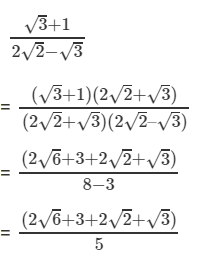(vii) Multiply and divide given number by 6 – 4√2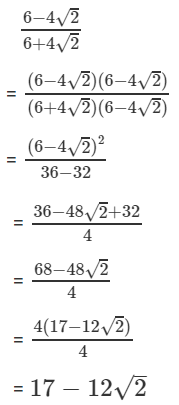(viii) Multiply and divide given number by 2√5 + 3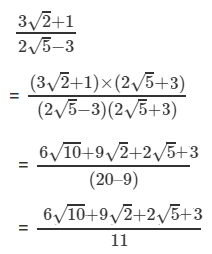(ix) Multiply and divide given number by √(a2+b2) – a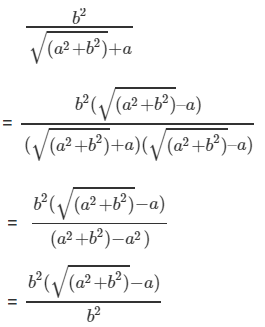Question 4: Rationales the denominator and simplify: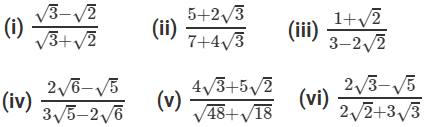Solution:

[Use identities: (a + b) (a – b) = a2 – b2 ; (a + b)2 = (a2 + 2ab + b2 and (a – b)2 = (a2 – 2ab + b2 ]

(i) Multiply both numerator and denominator by √3–√2 to rationalise the denominator.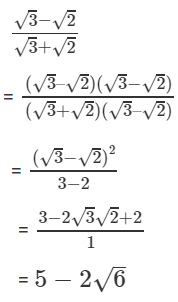(ii) Multiply both numerator and denominator by 7–4√3 to rationalise the denominator.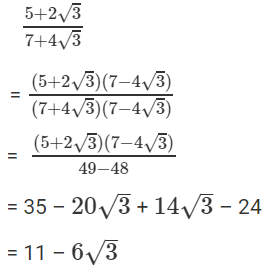(iii) Multiply both numerator and denominator by 3+2√2 to rationalise the denominator.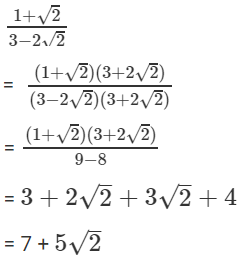(iv) Multiply both numerator and denominator by 3√5+2√6 to rationalise the denominator.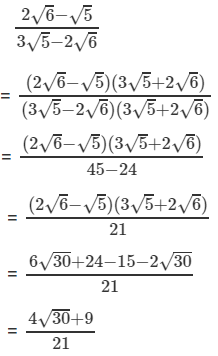(v) Multiply both numerator and denominator by √48–√18 to rationalise the denominator.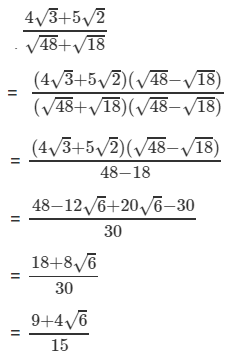(vi) Multiply both numerator and denominator by 2√2 – 3√3 to rationalise the denominator.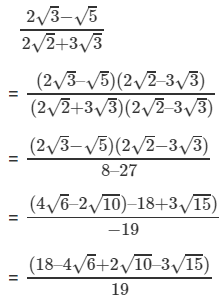## RD Sharma Solutions for Class 9 Maths Chapter 3 Rationalisation Exercise 3.2

RD Sharma Solutions Class 9 Maths Chapter 3 Rationalisation Exercise 3.2 is based on rationalisation of the denominator. In this process, we move a root from the bottom of a fraction to the top to simplify the fraction.